# Softmax function

The softmax function also referred to as softargmax:184 or normalized exponential, 198 may be a generalization of the logistic function to multiple dimensions.

#### Input

$$value=1$$ $$value=2$$ $$value=3$$ $$value=4$$ $$value=5$$

#### Solution

$$0.01165623095604$$ $$0.031684920796124$$ $$0.086128544436269$$ $$0.23412165725274$$ $$0.63640864655883$$

#### Formula

$$\normalsize Softmax\ function\ \sigma (\bf z \rm)_{j}$$ $$\\ \sigma (\bf z \rm)_{j} = \Large \frac{e^{z_{j}} }\sum_{k=1}^{K} e^{z_{k}} } \normalsize \hspace{3px} for\ j = 1,\cdots, K$$

## What is softmax function?

The softmax function also referred to as softargmax:184 or normalized exponential, 198 may be a generalization of the logistic function to multiple dimensions. It utilized in multinomial logistic regression and is usually used because the last activation function of a neural network to normalize the output of a network to a probability distribution over predicted output classes supported Luce's choice axiom.

The softmax function takes as input a vector z of K real numbers and normalizes it into a probability distribution consisting of K probabilities proportional to the exponentials of the input numbers. That is, before applying softmax, some vector components might be negative, or greater than one; and won't sum to 1; but after applying softmax, each component are going to be within the interval {\displaystyle (0,1)}(0,1), and therefore the components will add up to 1, in order that they will be interpreted as probabilities. Furthermore, the larger input components will correspond to larger probabilities.

The standard (unit) softmax function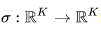is defined by the formula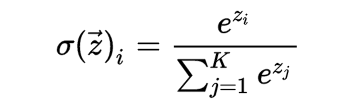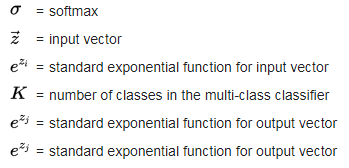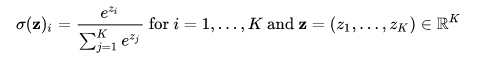In words: we apply the quality exponential to every element of the input vector and normalize these values by dividing by the sum of these exponentials; this normalization ensures that the sum of the components of the output vector is 1.

Instead of e, a special base b > 0 is often used; choosing a bigger value of b will create a probability distribution that's more concentrated around the positions of the most important input values. Writing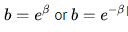yields the expressions: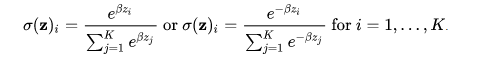In some fields, the bottom is fixed, like a hard and fast scale, while in others the parameter β is varied.

Using this tool is really good and it really helps full for you and these are the advantages of this tool.

While you use this tool you will be getting so many benefits from this tool.

This tool will save you time and you will be able to calculate is rapid.

You will not have to register or provide any kind of Emails to use this calculator.

You will be able to use this tool free there are no charges for use this.

You don’t even have to do it manually or use a formula to calculate any problem, it will automatically help you by just typing your value in here text boxes.

It is a really easy to use the tool so anyone can use it.

It is also available all over the world.

### How to use this tool softmax function

This tool is really easy to use and even our tool has a really very simple layout so that it will be easy to understand for people.

Now as you can see this tool doesn’t require any registration and it's a totally free online tool that anyone can use from anywhere. They can use it on the phone and they can use it on a desktop however they feel comfortable.

You can see on your screen you have only one text box in this tool right but you have another different number.

You must be confused about how you are going to type other numbers but you don’t need to worry too much because we have already mentioned how you will type numbers in them.

Just typing space or typing a comma at the end of each number will be fine.

And then you will be able to calculate your Function map single arrow.

Simply click on the calculate button to get the answer.

Tips: you should bookmark this tool so that you can use it in the future.

A.